# Banded matrix models¶

Computing the eigenvalues of a full $$N\times N$$ random matrix is $$\mathcal{O}(N^3)$$, and can thus become prohibitive for large $$N$$. A way to circumvent the problem is to adopt the equivalent banded models i.e. diagonalize banded matrices.

The first tridiagonal models for the Hermite Ensemble and Laguerre Ensemble were revealed by [DE02], who left the Jacobi Ensemble as an open question, addressed by [KN04]. Such tridiagonal formulations made sampling possible at cost $$\mathcal{O}(N^2)$$ but also unlocked sampling for generic $$\beta>0$$!

Note that [KN04] also derived a quindiagonal model for the Circular Ensemble.

## Hermite Ensemble¶

Take for reference measure $$\mu=\mathcal{N}(\mu, \sigma)$$

$(x_1,\dots,x_N) \sim \left|\Delta(x_1,\dots,x_N)\right|^{\beta} \prod_{i= 1}^N e^{- \frac{(x_i-\mu)^2}{2\sigma^2}} % \indic_{\bbR}(x_i) \ d x_i.$

Note

Recall that from the definition in (33)

$\left|\Delta(x_1,\dots,x_N)\right| = \prod_{i<j} |x_i - x_j|.$

$\begin{split}\begin{bmatrix} \alpha_1 & \sqrt{\beta_2}& 0 & 0 & 0 \\ \sqrt{\beta_2} & \alpha_2 & \sqrt{\beta_3}& 0 & 0 \\ 0 & \ddots & \ddots & \ddots & 0 \\ 0 & 0 & \sqrt{\beta_{N-1}} & \alpha_{N- 1} & \sqrt{\beta_{N}} \\ 0 & 0 & 0 & \sqrt{\beta_N} & \alpha_{N} \end{bmatrix},\end{split}$

with

$\alpha_{i} \sim \mathcal{N}(\mu, \sigma^2) \quad \text{and} \quad \beta_{i+1} \sim \Gamma\left(\frac{\beta}{2}(N - i), \sigma^2\right).$

To recover the full matrix model for Hermite Ensemble, recall that $$\Gamma(\frac{k}{2}, 2)\equiv \chi_k^2$$ and take

$\mu = 0 \quad \text{and} \quad \sigma^2 = 2.$

That is to say,

$\alpha_{i} \sim \mathcal{N}(0, 2) \quad \text{and} \quad \beta_{i+1} \sim \chi_{\beta(N - i)}^2.$
from dppy.beta_ensembles import HermiteEnsemble

hermite = HermiteEnsemble(beta=5.43)  # beta can be >=0, default beta=2
# Reference measure is N(mu, sigma^2)
hermite.sample_banded_model(loc=0.0, scale=1.0, size_N=500)
# hermite.plot(normalization=True)
hermite.hist(normalization=True)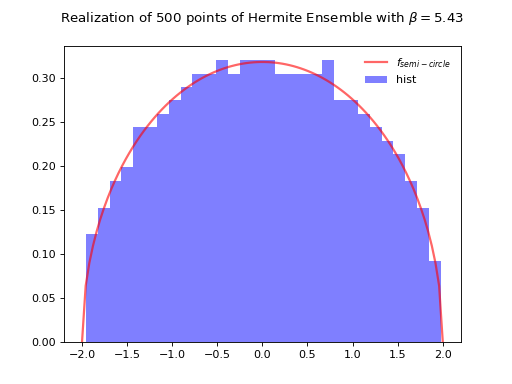Fig. 26 Tridiagonal matrix model for the Hermite ensemble

## Laguerre Ensemble¶

Take for reference measure $$\mu=\Gamma(k,\theta)$$

$(x_1,\dots,x_N) \sim \left|\Delta(x_1,\dots,x_N)\right|^{\beta} % \prod_{i= 1}^N x_i^{k-1} e^{- \frac{x_i}{\theta}} % \indic_{\bbR}(x_i) \ d x_i.$

Note

Recall that from the definition in (33)

$\left|\Delta(x_1,\dots,x_N)\right| = \prod_{i<j} |x_i - x_j|.$

$\begin{split}\begin{bmatrix} \alpha_1 & \sqrt{\beta_2}& 0 & 0 & 0 \\ \sqrt{\beta_2} & \alpha_2 & \sqrt{\beta_3}& 0 & 0 \\ 0 & \ddots & \ddots & \ddots & 0 \\ 0 & 0 & \sqrt{\beta_{N-1}} & \alpha_{N- 1} & \sqrt{\beta_{N}} \\ 0 & 0 & 0 & \sqrt{\beta_N} & \alpha_{N} \end{bmatrix} = \begin{bmatrix} \sqrt{\xi_1} & & & \\ \sqrt{\xi_2} & \sqrt{\xi_3} & & \\ & \ddots & \ddots & \\ & & \sqrt{\xi_{2N-2}} & \sqrt{\xi_{2N-1}} \end{bmatrix} \begin{bmatrix} \sqrt{\xi_1} & \sqrt{\xi_2} & & \\ & \sqrt{\xi_3} & \ddots & \\ & & \ddots & \sqrt{\xi_{2N-2}} \\ & & & \sqrt{\xi_{2N-1}} \end{bmatrix},\end{split}$

with

$\xi_{2i-1} \sim \Gamma\left(\frac{\beta}{2}(N - i) + k, \theta \right) \quad \text{and} \quad \xi_{2i} \sim \Gamma\left(\frac{\beta}{2}(N - i), \theta \right).$

To recover the full matrix model for Laguerre Ensemble, recall that $$\Gamma(\frac{k}{2}, 2)\equiv \chi_k^2$$ and take

$k = \frac{\beta}{2}(M-N+1) \quad \text{and} \quad \theta = 2.$

That is to say,

$\xi_{2i-1} \sim \chi_{\beta(M - i + 1)}^2 \quad \text{and} \quad \xi_{2i} \sim \chi_{\beta(N - i)}^2.$
from dppy.beta_ensembles import LaguerreEnsemble

laguerre = LaguerreEnsemble(beta=2.98)  # beta can be >=0, default beta=2
# Reference measure is Gamma(k, theta)
laguerre.sample_banded_model(shape=600, scale=2.0, size_N=400)
# laguerre.plot(normalization=True)
laguerre.hist(normalization=True)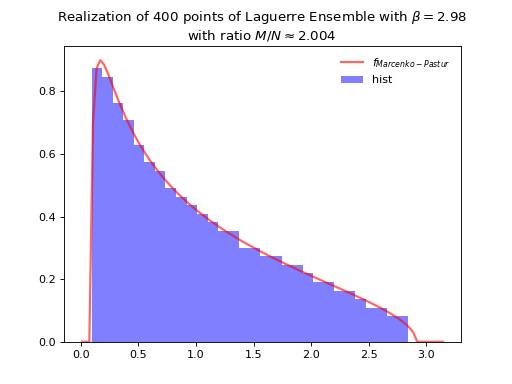Fig. 28 Tridiagonal matrix model for the Laguerre ensemble

## Jacobi Ensemble¶

Take for reference measure $$\mu=\operatorname{Beta}(a,b)$$

$(x_1,\dots,x_N) \sim \left|\Delta(x_1,\dots,x_N)\right|^{\beta} % \prod_{i= 1}^N x_i^{a-1} (1-x_i)^{b-1} % \indic_{\bbR}(x_i) \ d x_i.$

Note

Recall that from the definition in (33)

$\left|\Delta(x_1,\dots,x_N)\right| = \prod_{i<j} |x_i - x_j|.$

$\begin{split}\begin{bmatrix} \alpha_1 & \sqrt{\beta_2}& 0 & 0 & 0 \\ \sqrt{\beta_2} & \alpha_2 & \sqrt{\beta_3}& 0 & 0 \\ 0 & \ddots & \ddots & \ddots & 0 \\ 0 & 0 & \sqrt{\beta_{N-1}} & \alpha_{N- 1} & \sqrt{\beta_{N}} \\ 0 & 0 & 0 & \sqrt{\beta_N} & \alpha_{N} \end{bmatrix}.\end{split}$
\begin{align}\begin{aligned}\begin{split}\begin{aligned} \alpha_1 &= \xi_1 \quad & \\ \alpha_k &= \xi_{2k-2}+\xi_{2k-1} \quad &\beta_{k+1} &= \xi_{2k-1}\xi_{2k} \end{aligned}\end{split}\\\begin{split}\begin{aligned} \xi_1 &= c_1 \quad &\gamma_1 &= 1-c_1 \\ \xi_k &= (1-c_{k-1})c_k \quad &\gamma_k &= c_{k-1}(1-c_k) \end{aligned},\end{split}\end{aligned}\end{align}

with

$c_{2i-1} \sim \operatorname{Beta} \left( \frac{\beta}{2}(N-i) + a, \frac{\beta}{2}(N-i) + b \right) \quad \text{and} \quad c_{2i} \sim \operatorname{Beta} \left( \frac{\beta}{2} (N-i), \frac{\beta}{2} (N-i-1) + a + b \right).$

To recover the full matrix model for Laguerre Ensemble, recall that $$\Gamma(\frac{k}{2}, 2)\equiv \chi_k^2$$ and take

$a = \frac{\beta}{2}(M_1-N+1) \quad \text{and} \quad b = \frac{\beta}{2}(M_2-N+1).$

That is to say,

$c_{2i-1} \sim \operatorname{Beta} \left( \frac{\beta}{2}(M_1-i+1), \frac{\beta}{2}(M_2-i+1) \right) \quad \text{and} \quad c_{2i} \sim \operatorname{Beta} \left( \frac{\beta}{2} (N-i), \frac{\beta}{2} (M_1+M_2-N-i+1) \right).$
from dppy.beta_ensembles import JacobiEnsemble

jacobi = JacobiEnsemble(beta=3.14)  # beta can be >=0, default beta=2
# Reference measure is Beta(a,b)
jacobi.sample_banded_model(a=500, b=300, size_N=400)
# jacobi.plot(normalization=True)
jacobi.hist(normalization=True)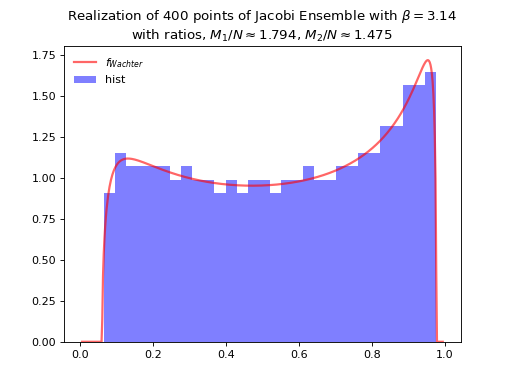Fig. 30 Tridiagonal matrix model for the Jacobi ensemble

## Circular Ensemble¶

$\left|\Delta(e^{i \theta_1},\dots, e^{i \theta_N})\right|^{\beta} \prod_{j = 1}^N \frac{1}{2\pi} \mathbf{1}_{[0,2\pi]} (\theta_j) d\theta_j.$

Note

Recall that from the definition in (33)

$\left|\Delta(x_1,\dots,x_N)\right| = \prod_{i<j} |x_i - x_j|.$

Important

Consider the distribution $$\Theta_{\nu}$$ that for integers $$\nu\geq2$$ is defined as follows:

Draw $$v$$ uniformly at random from the unit sphere $$\mathbb{S}^{\nu} \in \mathbb{R}^{\nu+1}$$, then $$v_1 + iv_2\sim \Theta_{\nu}$$

Now, given $$\beta\in \mathbb{N}^*$$, let

• $$\alpha_k\sim \Theta_{\beta(N-k-1)+1}$$ independent variables

• for $$0\leq k\leq N-1$$ set $$\rho_k = \sqrt{1-|\alpha_k|^2}$$.

Then, the equivalent quindiagonal model corresponds to the eigenvalues of either $$LM$$ or $$ML$$ with

$L = \operatorname{diag}[\Xi_0,\Xi_2,\dots] \quad \text{and} \quad M = \operatorname{diag}[\Xi_{-1},\Xi_1,\Xi_3\dots],$

and where

$\begin{split}\Xi_k = \begin{bmatrix} \overline{\alpha}_k & \rho_k\\ \rho_k & -\alpha_k \end{bmatrix} , \quad 0\leq k\leq N-2 , \quad \text{with} \quad \Xi_{-1} =  \quad \text{and} \quad \Xi_{N-1} = [\overline{\alpha}_{N-1}].\end{split}$

Hint

The effect of increasing the $$\beta$$ parameter can be nicely visualized on this Circular Ensemble. Viewing $$\beta$$ as the inverse temperature, the configuration of the eigenvalues crystallizes with $$\beta$$, see the figure below.

from dppy.beta_ensembles import CircularEnsemble

circular = CircularEnsemble(beta=2)  # beta must be >=0 integer, default beta=2

# See the cristallization of the configuration as beta increases
for b in [0, 1, 5, 10]:

circular.beta = b
circular.sample_banded_model(size_N=30)
circular.plot()

circular.beta = 2
circular.sample_banded_model(size_N=1000)
circular.hist()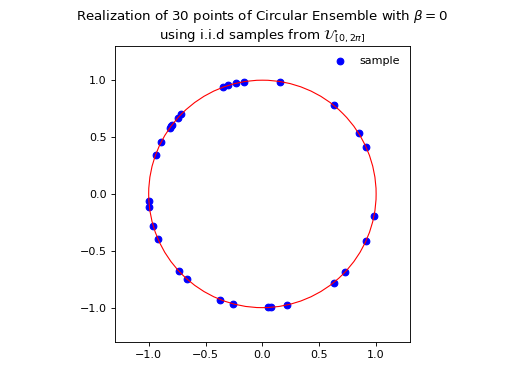Fig. 32 (png, hires.png, pdf) Quindiagonal matrix model for the Circular ensemble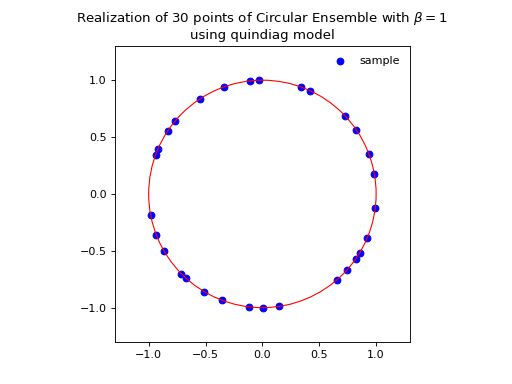Fig. 33 (png, hires.png, pdf) Quindiagonal matrix model for the Circular ensemble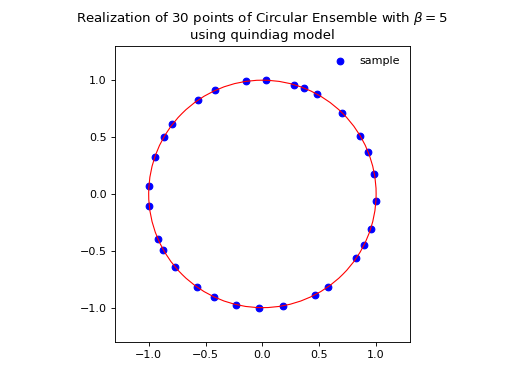Fig. 34 (png, hires.png, pdf) Quindiagonal matrix model for the Circular ensemble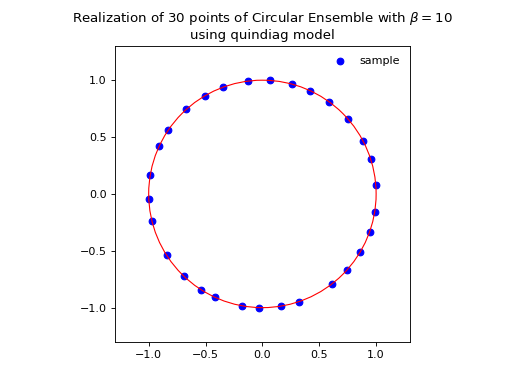Fig. 35 (png, hires.png, pdf) Quindiagonal matrix model for the Circular ensemble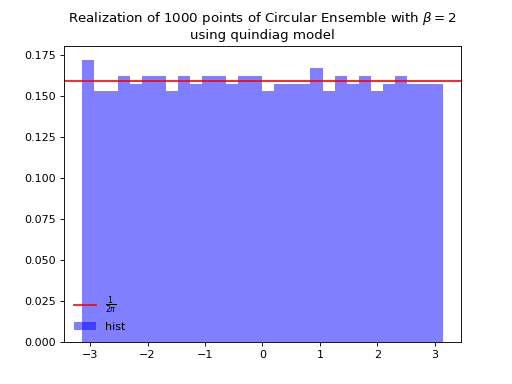Fig. 36 (png, hires.png, pdf) Quindiagonal matrix model for the Circular ensemble